# Are for-loops in pandas really bad? When should I care?

Are `for` loops really “bad”? If not, in what situation(s) would they be better than using a more conventional “vectorized” approach?1

I am familiar with the concept of “vectorization”, and how pandas employs vectorized techniques to speed up computation. Vectorized functions broadcast operations over the entire series or DataFrame to achieve speedups much greater than conventionally iterating over the data.

However, I am quite surprised to see a lot of code (including from answers on Stack Overflow) offering solutions to problems that involve looping through data using `for` loops and list comprehensions. The documentation and API say that loops are “bad”, and that one should “never” iterate over arrays, series, or DataFrames. So, how come I sometimes see users suggesting loop-based solutions?

1 – While it is true that the question sounds somewhat broad, the truth is that there are very specific situations when `for` loops are usually better than conventionally iterating over data. This post aims to capture this for posterity.

### Method 1

TLDR; No, `for` loops are not blanket “bad”, at least, not always. It is probably more accurate to say that some vectorized operations are slower than iterating, versus saying that iteration is faster than some vectorized operations. Knowing when and why is key to getting the most performance out of your code. In a nutshell, these are the situations where it is worth considering an alternative to vectorized pandas functions:

1. When your data is small (…depending on what you’re doing),
2. When dealing with `object`/mixed dtypes
3. When using the `str`/regex accessor functions

Let’s examine these situations individually.

#### Iteration v/s Vectorization on Small Data

Pandas follows a “Convention Over Configuration” approach in its API design. This means that the same API has been fitted to cater to a broad range of data and use cases.

When a pandas function is called, the following things (among others) must internally be handled by the function, to ensure working

1. Index/axis alignment
2. Handling mixed datatypes
3. Handling missing data

Almost every function will have to deal with these to varying extents, and this presents an overhead. The overhead is less for numeric functions (for example, `Series.add`), while it is more pronounced for string functions (for example, `Series.str.replace`).

`for` loops, on the other hand, are faster then you think. What’s even better is list comprehensions (which create lists through `for` loops) are even faster as they are optimized iterative mechanisms for list creation.

`[f(x) for x in seq]`

Where `seq` is a pandas series or DataFrame column. Or, when operating over multiple columns,

`[f(x, y) for x, y in zip(seq1, seq2)]`

Where `seq1` and `seq2` are columns.

Numeric Comparison
Consider a simple boolean indexing operation. The list comprehension method has been timed against `Series.ne` (`!=`) and `query`. Here are the functions:

```# Boolean indexing with Numeric value comparison.
df[df.A != df.B]                            # vectorized !=
df.query('A != B')                          # query (numexpr)
df[[x != y for x, y in zip(df.A, df.B)]]    # list comp```

For simplicity, I have used the `perfplot` package to run all the timeit tests in this post. The timings for the operations above are below:The list comprehension outperforms `query` for moderately sized N, and even outperforms the vectorized not equals comparison for tiny N. Unfortunately, the list comprehension scales linearly, so it does not offer much performance gain for larger N.

Note
It is worth mentioning that much of the benefit of list comprehension come from not having to worry about the index alignment,
but this means that if your code is dependent on indexing alignment,
this will break. In some cases, vectorised operations over the
underlying NumPy arrays can be considered as bringing in the “best of
both worlds”, allowing for vectorisation without all the unneeded overhead of the pandas functions. This means that you can rewrite the operation above as

`df[df.A.values != df.B.values]`

Which outperforms both the pandas and list comprehension equivalents: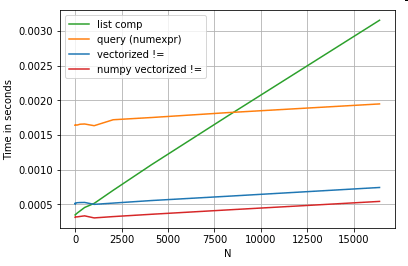NumPy vectorization is out of the scope of this post, but it is definitely worth considering, if performance matters.

Value Counts
Taking another example – this time, with another vanilla python construct that is faster than a for loop – `collections.Counter`. A common requirement is to compute the value counts and return the result as a dictionary. This is done with `value_counts`, `np.unique`, and `Counter`:

```# Value Counts comparison.
ser.value_counts(sort=False).to_dict()           # value_counts
dict(zip(*np.unique(ser, return_counts=True)))   # np.unique
Counter(ser)                                     # Counter```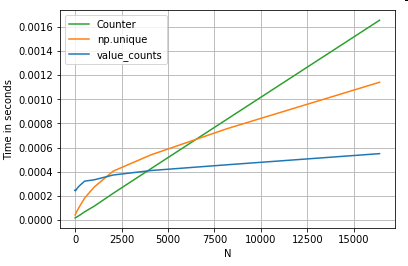The results are more pronounced, `Counter` wins out over both vectorized methods for a larger range of small N (~3500).

Note
More trivia (courtesy @user2357112). The `Counter` is implemented with a C
accelerator
,
so while it still has to work with python objects instead of the
underlying C datatypes, it is still faster than a `for` loop. Python
power!

Of course, the take away from here is that the performance depends on your data and use case. The point of these examples is to convince you not to rule out these solutions as legitimate options. If these still don’t give you the performance you need, there is always cython and numba. Let’s add this test into the mix.

```from numba import njit, prange

@njit(parallel=True)
result = [False] * len(x)
for i in prange(len(x)):
result[i] = x[i] != y[i]

return np.array(result)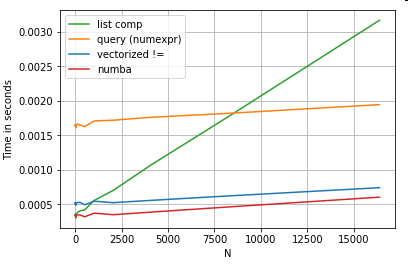Numba offers JIT compilation of loopy python code to very powerful vectorized code. Understanding how to make numba work involves a learning curve.

#### Operations with Mixed/`object` dtypes

String-based Comparison
Revisiting the filtering example from the first section, what if the columns being compared are strings? Consider the same 3 functions above, but with the input DataFrame cast to string.

```# Boolean indexing with string value comparison.
df[df.A != df.B]                            # vectorized !=
df.query('A != B')                          # query (numexpr)
df[[x != y for x, y in zip(df.A, df.B)]]    # list comp```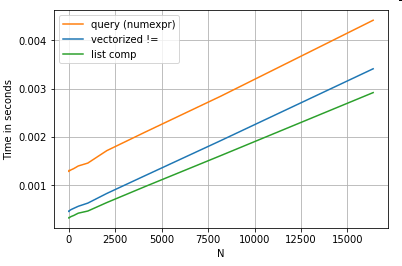So, what changed? The thing to note here is that string operations are inherently difficult to vectorize. Pandas treats strings as objects, and all operations on objects fall back to a slow, loopy implementation.

Now, because this loopy implementation is surrounded by all the overhead mentioned above, there is a constant magnitude difference between these solutions, even though they scale the same.

When it comes to operations on mutable/complex objects, there is no comparison. List comprehension outperforms all operations involving dicts and lists.

Accessing Dictionary Value(s) by Key
Here are timings for two operations that extract a value from a column of dictionaries: `map` and the list comprehension. The setup is in the Appendix, under the heading “Code Snippets”.

```# Dictionary value extraction.
ser.map(operator.itemgetter('value'))     # map
pd.Series([x.get('value') for x in ser])  # list comprehension```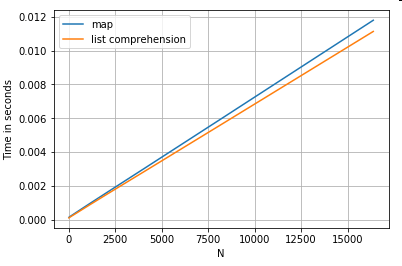Positional List Indexing
Timings for 3 operations that extract the 0th element from a list of columns (handling exceptions), `map`, `str.get` accessor method, and the list comprehension:

``````# List positional indexing.
def get_0th(lst):
try:
return lst
# Handle empty lists and NaNs gracefully.
except (IndexError, TypeError):
return np.nan
``````
```ser.map(get_0th)                                          # map
ser.str                                                # str accessor
pd.Series([x if len(x) > 0 else np.nan for x in ser])  # list comp
pd.Series([get_0th(x) for x in ser])                      # list comp safe```

Note
If the index matters, you would want to do:

`pd.Series([...], index=ser.index)`

When reconstructing the series.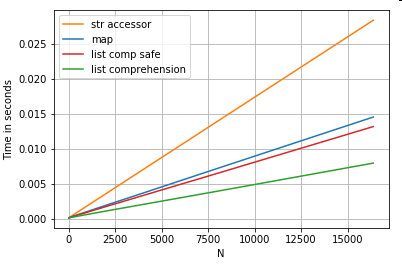List Flattening
A final example is flattening lists. This is another common problem, and demonstrates just how powerful pure python is here.

```# Nested list flattening.
pd.DataFrame(ser.tolist()).stack().reset_index(drop=True)  # stack
pd.Series(list(chain.from_iterable(ser.tolist())))         # itertools.chain
pd.Series([y for x in ser for y in x])                     # nested list comp```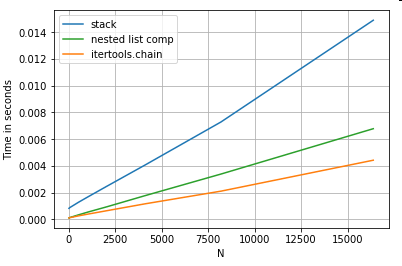Both `itertools.chain.from_iterable` and the nested list comprehension are pure python constructs, and scale much better than the `stack` solution.

These timings are a strong indication of the fact that pandas is not equipped to work with mixed dtypes, and that you should probably refrain from using it to do so. Wherever possible, data should be present as scalar values (ints/floats/strings) in separate columns.

Lastly, the applicability of these solutions depend widely on your data. So, the best thing to do would be to test these operations on your data before deciding what to go with. Notice how I have not timed `apply` on these solutions, because it would skew the graph (yes, it’s that slow).

#### Regex Operations, and `.str` Accessor Methods

Pandas can apply regex operations such as `str.contains`, `str.extract`, and `str.extractall`, as well as other “vectorized” string operations (such as `str.split`, `str.find`, `str.translate`, and so on) on string columns. These functions are slower than list comprehensions, and are meant to be more convenience functions than anything else.

It is usually much faster to pre-compile a regex pattern and iterate over your data with `re.compile` (also see Is it worth using Python’s re.compile?). The list comp equivalent to `str.contains` looks something like this:

```p = re.compile(...)
ser2 = pd.Series([x for x in ser if p.search(x)])```

Or,

`ser2 = ser[[bool(p.search(x)) for x in ser]]`

If you need to handle NaNs, you can do something like

`ser[[bool(p.search(x)) if pd.notnull(x) else False for x in ser]]`

The list comp equivalent to `str.extract` (without groups) will look something like:

`df['col2'] = [p.search(x).group(0) for x in df['col']]`

If you need to handle no-matches and NaNs, you can use a custom function (still faster!):

```def matcher(x):
m = p.search(str(x))
if m:
return m.group(0)
return np.nan

df['col2'] = [matcher(x) for x in df['col']]```

The `matcher` function is very extensible. It can be fitted to return a list for each capture group, as needed. Just extract query the `group` or `groups` attribute of the matcher object.

For `str.extractall`, change `p.search` to `p.findall`.

String Extraction
Consider a simple filtering operation. The idea is to extract 4 digits if it is preceded by an upper case letter.

```# Extracting strings.
p = re.compile(r'(?<=[A-Z])(d{4})')
def matcher(x):
m = p.search(x)
if m:
return m.group(0)
return np.nan

ser.str.extract(r'(?<=[A-Z])(d{4})', expand=False)   #  str.extract
pd.Series([matcher(x) for x in ser])                  #  list comprehension```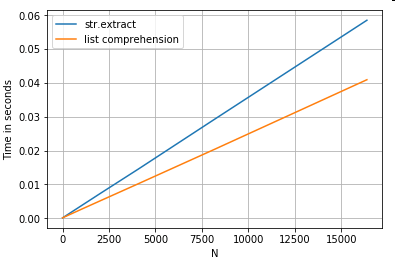More Examples
Full disclosure – I am the author (in part or whole) of these posts listed below.

#### Conclusion

As shown from the examples above, iteration shines when working with small rows of DataFrames, mixed datatypes, and regular expressions.

The speedup you get depends on your data and your problem, so your mileage may vary. The best thing to do is to carefully run tests and see if the payout is worth the effort.

The “vectorized” functions shine in their simplicity and readability, so if performance is not critical, you should definitely prefer those.

Another side note, certain string operations deal with constraints that favour the use of NumPy. Here are two examples where careful NumPy vectorization outperforms python:

Additionally, sometimes just operating on the underlying arrays via `.values` as opposed to on the Series or DataFrames can offer a healthy enough speedup for most usual scenarios (see the Note in the Numeric Comparison section above). So, for example `df[df.A.values != df.B.values]` would show instant performance boosts over `df[df.A != df.B]`. Using `.values` may not be appropriate in every situation, but it is a useful hack to know.

As mentioned above, it’s up to you to decide whether these solutions are worth the trouble of implementing.

#### Appendix: Code Snippets

```import perfplot
import operator
import pandas as pd
import numpy as np
import re

from collections import Counter
from itertools import chain```

<!- ->

```# Boolean indexing with Numeric value comparison.
perfplot.show(
setup=lambda n: pd.DataFrame(np.random.choice(1000, (n, 2)), columns=['A','B']),
kernels=[
lambda df: df[df.A != df.B],
lambda df: df.query('A != B'),
lambda df: df[[x != y for x, y in zip(df.A, df.B)]],
],
labels=['vectorized !=', 'query (numexpr)', 'list comp', 'numba'],
n_range=[2**k for k in range(0, 15)],
xlabel='N'
)```

<!- ->

```# Value Counts comparison.
perfplot.show(
setup=lambda n: pd.Series(np.random.choice(1000, n)),
kernels=[
lambda ser: ser.value_counts(sort=False).to_dict(),
lambda ser: dict(zip(*np.unique(ser, return_counts=True))),
lambda ser: Counter(ser),
],
labels=['value_counts', 'np.unique', 'Counter'],
n_range=[2**k for k in range(0, 15)],
xlabel='N',
equality_check=lambda x, y: dict(x) == dict(y)
)```

<!- ->

```# Boolean indexing with string value comparison.
perfplot.show(
setup=lambda n: pd.DataFrame(np.random.choice(1000, (n, 2)), columns=['A','B'], dtype=str),
kernels=[
lambda df: df[df.A != df.B],
lambda df: df.query('A != B'),
lambda df: df[[x != y for x, y in zip(df.A, df.B)]],
],
labels=['vectorized !=', 'query (numexpr)', 'list comp'],
n_range=[2**k for k in range(0, 15)],
xlabel='N',
equality_check=None
)```

<!- ->

```# Dictionary value extraction.
ser1 = pd.Series([{'key': 'abc', 'value': 123}, {'key': 'xyz', 'value': 456}])
perfplot.show(
setup=lambda n: pd.concat([ser1] * n, ignore_index=True),
kernels=[
lambda ser: ser.map(operator.itemgetter('value')),
lambda ser: pd.Series([x.get('value') for x in ser]),
],
labels=['map', 'list comprehension'],
n_range=[2**k for k in range(0, 15)],
xlabel='N',
equality_check=None
)```

<!- ->

```# List positional indexing.
ser2 = pd.Series([['a', 'b', 'c'], [1, 2], []])
perfplot.show(
setup=lambda n: pd.concat([ser2] * n, ignore_index=True),
kernels=[
lambda ser: ser.map(get_0th),
lambda ser: ser.str,
lambda ser: pd.Series([x if len(x) > 0 else np.nan for x in ser]),
lambda ser: pd.Series([get_0th(x) for x in ser]),
],
labels=['map', 'str accessor', 'list comprehension', 'list comp safe'],
n_range=[2**k for k in range(0, 15)],
xlabel='N',
equality_check=None
)```

<!- ->

```# Nested list flattening.
ser3 = pd.Series([['a', 'b', 'c'], ['d', 'e'], ['f', 'g']])
perfplot.show(
setup=lambda n: pd.concat([ser2] * n, ignore_index=True),
kernels=[
lambda ser: pd.DataFrame(ser.tolist()).stack().reset_index(drop=True),
lambda ser: pd.Series(list(chain.from_iterable(ser.tolist()))),
lambda ser: pd.Series([y for x in ser for y in x]),
],
labels=['stack', 'itertools.chain', 'nested list comp'],
n_range=[2**k for k in range(0, 15)],
xlabel='N',
equality_check=None

)```

<!- _>

```# Extracting strings.
ser4 = pd.Series(['foo xyz', 'test A1234', 'D3345 xtz'])
perfplot.show(
setup=lambda n: pd.concat([ser4] * n, ignore_index=True),
kernels=[
lambda ser: ser.str.extract(r'(?<=[A-Z])(d{4})', expand=False),
lambda ser: pd.Series([matcher(x) for x in ser])
],
labels=['str.extract', 'list comprehension'],
n_range=[2**k for k in range(0, 15)],
xlabel='N',
equality_check=None
)```

### Method 2

In short

• for loop + `iterrows` is extremely slow. Overhead isn’t significant on ~1k rows, but noticeable on 10k+ rows.
• for loop + `itertuples` is much faster than `iterrows` or `apply`.
• vectorization is usually much faster than `itertuples`